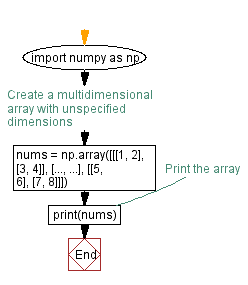﻿ Creating multidimensional arrays with unspecified dimensions in Python

# Python: Creating multidimensional arrays with unspecified dimensions

## Python ellipsis (...) Data Type: Exercise-6 with Solution

Write a Python program that uses 'Ellipsis' to create a multidimensional array with unspecified dimensions.

Sample Solution:

Code:

``````import numpy as np
# Create a multidimensional array with unspecified dimensions
nums = np.array([[[1, 2], [3, 4]], [..., ...], [[5, 6], [7, 8]]])
# Print the array
print(nums)
``````

Output:

```[[list([1, 2]) list([3, 4])]
[Ellipsis Ellipsis]
[list([5, 6]) list([7, 8])]]
```

In the exercise above, we use 'ellipsis (...)' to represent unspecified dimensions in a NumPy array. The resulting array contains 'ellipsis' as placeholders for dimensions not explicitly specified. In this way, we can create multidimensional arrays without having to specify all dimensions in advance.

Flowchart:What is the difficulty level of this exercise?

Test your Programming skills with w3resource's quiz.

﻿

## Python: Tips of the Day

Summing a sequence of numbers (calculating the sum of zero to ten with skips):

```>>> l = range(0,10,2)
>>> sum(l)
20
```

We are closing our Disqus commenting system for some maintenanace issues. You may write to us at reach[at]yahoo[dot]com or visit us at Facebook